Welcome To Basics In Maths

# Product of Vectors (Qns.& Ans) V.S.A.Q.’S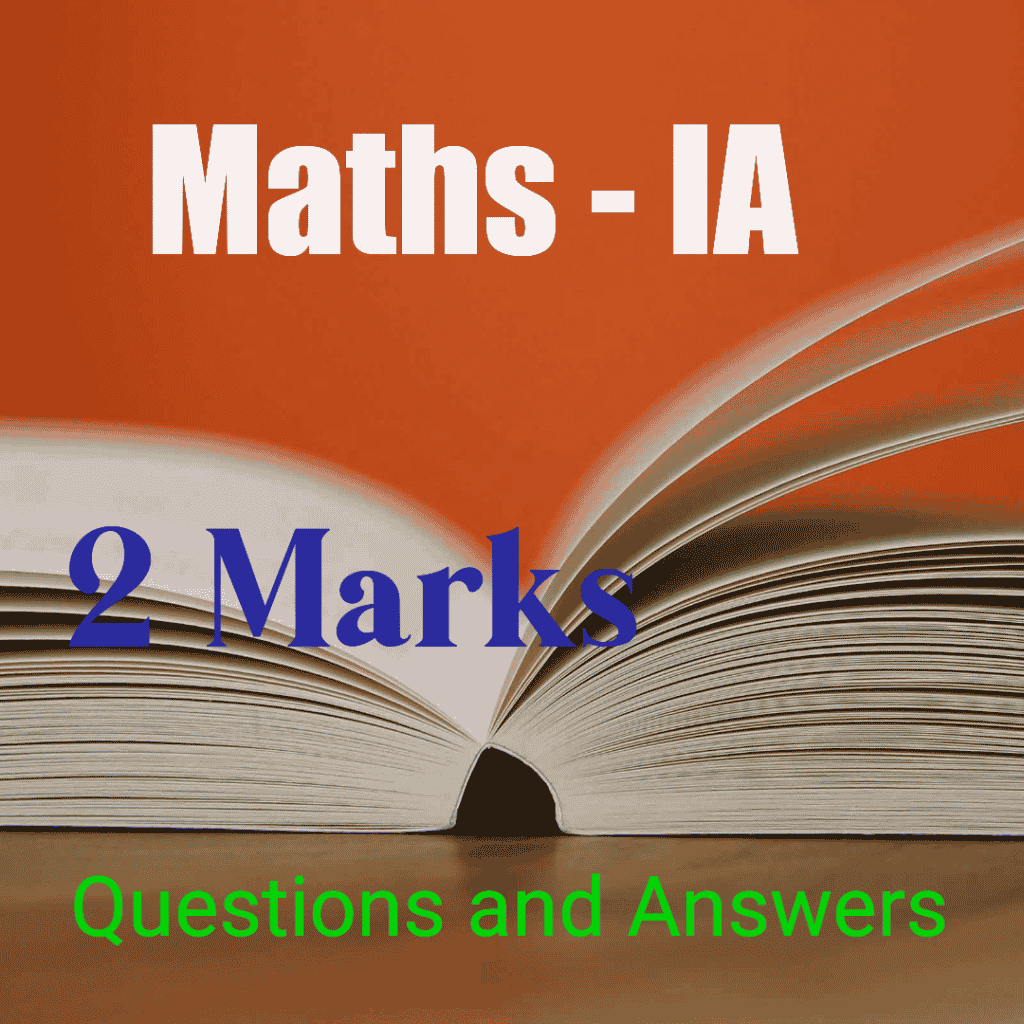# These solutions designed by the ‘Basics in Maths‘ team. These notes to do help the intermediate First-year Maths students.

### Product Of Vectors

Question 1

If a = 6i +2 j +3 k, b = 2i – 9 j+ 6k, then find the angle between the vectors a and b

Sol:

Given vectors are a = 6i +2 j +3 k, b = 2i – 9 j+ 6k

If θ is the angle between the vectors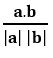a and b, then cos θ =

a .b = (6i +2 j +3 k). (2i – 9 j+ 6k) = 6(2) + 2 (– 9) + 3(6)

= 12 – 18 + 18 = 12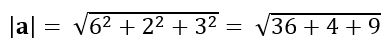= 7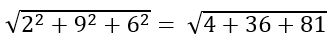= 11

Question 2

If a = i +2 j –3 k, b = 3ij+ 2k, then show that a + b and ab are perpendicular to each other.

Sol:

Given vectors are a = i +2 j –3 k, b = 3ij+ 2k

a + b = (i +2 j –3 k) + (3ij+ 2k) = 4i + jk

ab = (i +2 j –3 k) – (3ij+ 2k) = –2i +3 j – 5k

(a + b). (ab) = (4i + jk). (–2i +3 j – 5k)

= – 8 + 3 + 5

= 0

a + b and ab is perpendicular to each other.

### Question 3

If a and b be non-zero, non-collinear vectors. If , then find the angle between a and b

Sol:

Squaring on both sides

(a + b) (a + b) = (a – b) (a – b)

a2 + 2 a. b + b2 = a2 – 2 a.b + b2

⟹ 4 a.b = 0

a.b = 0

∴ the angle between a and b  is 900

### Question 4

Sol:

(11)2 2 ×11×23 cos θ + (23)2 = 900

121 506 cos θ + 529   = 900

650 506 cos θ = 900

= (11)2 + 2 ×11×23 cos θ + (23)2

= 121 + 2 ×11×23 ×  + 529

= 400

### Question 5

If a = ijk and b = 2i – 3j + k, then find the projection vector of b on a and its magnitude.

Sol:

Given vectors are a = ijk and b = 2i – 3j + k

a.b = (ij k). (2i – 3j + k) = 2 + 3 – 1 = 4

The projection vector of b on a =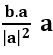### Question 6

If the vectors λ i – 3j + 5k and 2λ i – λ jk are perpendicular to each other, then find λ

Sol:

let a = λ i – 3j + 5k, b = 2λ i – λ jk

Given, that a and b are perpendicular to each other

a.b = 0

i – 3j + 5k). (2λ i – λ jk) = 0

2 λ2 + 3 λ – 5 = 0

2 λ2 + 5 λ – 2 λ – 5 = 0

λ (2 λ + 5) – 1 (2 λ + 5) = 0

(2 λ + 5) ((λ – 1) = 0

λ = 1 or λ = -5/2

### Question 7

Find the Cartesian equation of the plane passing through the point (– 2, 1, 3) and perpendicular to the vector 3i + j + 5k

Sol:

let P (x, y, z) be any point on the plane

⟹ OP = xi + yj + zk

OA = – 2i +j +3k

AP = OPOA = (xi + yj + zk) – (– 2i +j +3k)

AP = (x + 2) i + (y – 1) j + (z – 3) k

AP is perpendicular to the vector 3i + j + 5k

⟹ 3 (x + 2) + (y – 1) + 5(z – 3) = 0

⟹ 3x + 6 + y – 1 + 5z – 15 = 0

∴   3x + y + 5z – 10 = 0 is the required Cartesian equation of the plane

### Question 8

Find the angle between the planes 2x – 3y – 6z = 5 and 6x + 2y – 9z = 4

Sol:

Given plane equations are: 2x – 3y – 6z = 5,6x + 2y – 9z = 4

Vector equations of the above planes are: r. (2i – 3j – 6k) = 5 and r. (6i + 2j – 9k) = 4

⟹ n1 = 2i – 3j – 6k and n2 = 6i + 2j – 9k

If θ is the angle between the planes r. n1 = d1 and r. n2 = d2, then

### Question 9

a = 2ij + k, b = i – 3j – 5k. Find the vector c such that a, b, and c form the sides of a triangle.

Sol:

Given a = 2ij + k, b = i – 3j – 5k

If a, b, and c form the sides of a triangle, then a + b + c = 0

⟹ a + b = – c

c = – (a + b)

= – [(2ij + k) +( i – 3j – 5k)]

= – (3i –4 j –4k)

∴ c = – 3i +4 j + 4k

### Question 10

Find the equation of the plane through the point (3, –2, 1) and perpendicular to the vector (4, 7, –4).

Sol:

Let a = 3i – 2j + k and n = 4i + 7j – 4k

The equation of the plane passing through point A(a) and perpendicular to the vector n is (ra). n = 0

⟹ [r – (3i – 2j + k)]. (4i + 7j – 4k) = 0

⟹ r. (4i + 7j – 4k)– [(3i – 2j + k). (4i + 7j – 4k)] = 0

r. (4i + 7j – 4k)– (12 – 14 – 4) = 0

r. (4i + 7j – 4k)– 6 = 0

r. (4i + 7j – 4k) = 6

### Question 11

Find the unit vector parallel to the XOY-plane and perpendicular to the vector 4i – 3j + k

Sol:

The vector which is parallel to the XOY-plane is of the form xi + yj

The vector which is parallel to the XOY-plane and perpendicular to 4i – 3j + k

is 3i + 4j

∴ The unit vector parallel to the XOY-plane and perpendicular to the vector 4i – 3j + k =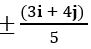### Question 12

Sol:

Given, a + b + c = 0,  = 3,  = 5 and    = 7

a + b = – c

32 + 52 + 2  cos θ = 72

9 + 25 + 2.3.5 cos θ = 49

34 + 30cos θ = 49

30cos θ = 49 – 34

30cos θ = 15

cos θ = 15/30 = 1/2

∴ θ = π/3

### Question 13

If a = 2i – 3j + 5k, b = – i + 4j + 2k, then find a × b and unit vector perpendicular to both a and b

Sol:

Given, a = 2i – 3j + 5k, b = – i + 4j + 2k

= i (–6 – 20) – j (4 + 5) + k (8 – 3)

= –26i – 9j + 5k

The unit vector perpendicular to both a and b    =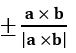### Question 14

If a = i + j + 2k and b = 3i + 5jk are two sides of a triangle, then find its area.

Sol:

Given, a = i + 2j + 3k and b = 3i + 5jk

If a, b are two sides of a triangle, then area of the triangle =

= i (–2 – 15) – j (–1 – 9) + k (5 – 6)

= –17i + 10 j k

### Question 15

Find the area of the parallelogram for which the vectors a = 2i – 3j and b = 3ik are adjacent sides.

Sol:

Given, a = 2i – 3j and b = 3ik are adjacent sides of a parallelogram

The area of the parallelogram whose vectors a , b are adjacent sides =

= i (3 – 0) – j (–2 – 0) + k (0 + 9)

=3 i +2 j +9 k

∴ The area of the parallelogram =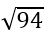### Question 16

Let a, b be two non-collinear unit vectors. If α = a – (a . b) b and β = a × b, then show that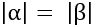Sol:

= 1 – cos2 θ

= sin2 θ

= 1 + cos2 θ – 2cos2 θ

= 1– cos2 θ

= sin2 θ

### Question 17

Sol:

Let a = xi + yj + zk

= i ( 0 – 0) – j (0 – z) + k (0 – y)

= – yk + zj

= 2(x2 + y2 +z2)

### Question 18

Sol:

= 2 × 3 sin

= 2 × 3×1/2

= 3

### Question 19

If 4i + j + pk is parallel to the vector i + 2j + 3k, then find p.

Sol:

Given 4i +  j + pk is parallel to the vector i + 2j + 3k

⇒ p = 12

### Question 20

Compute a× (b + c) + b× (c + a) + c× (a + b)

Sol:

a× (b + c) + b× (c + a) + c× (a + b)

= a× b + a× c + b× c + b× a + c × a + c × b

= a× b + a× c + b× ca × b a × c b ×c

= 0

### Question 21

Compute 2j× (3i – 4k) + (i + 2j) × k

Sol:

2j× (3i – 4k) + (i + 2j) × k

= 6(j × i) – 8(j × k) + (i × k) + 2(j × k)

= 6k – 8i – j + 2i

= 6i –j 6 j